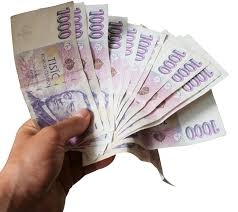# Deposited 7096

Mr. Novák deposited CZK 50,000 a year in the bank at an annual interest rate of 4%. How much will pre-tax interest be after one year?

u =  2000 Kc

### Step-by-step explanation:Did you find an error or inaccuracy? Feel free to write us. Thank you!

Tips for related online calculators
Need help calculating sum, simplifying, or multiplying fractions? Try our fraction calculator.
Our percentage calculator will help you quickly calculate various typical tasks with percentages.
Do you want to convert time units like minutes to seconds?